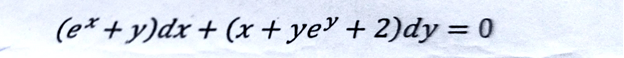# Differential equations past/Previous question paper

– Differential equations-Computer Science all subject past paper  -Differential equations   with source code – Differential equations  Course – Differential equations  idea -Differential equations MCQ – Differential equations  Interview Question  –Computer Science all courses -Technology –University Past Paper -Programming language –Question paperold paper

## Differential equations Mid Term Examination 2021

Q1: a) Verify that the indicated function is the solution of the given differential equation

1. b) Determine whether the given function is Homogenous or not?

If so, describe the degree of  homogeneity.

Q2:Use the method of undetermined co-efficient to solve the following differential equation.

Q3: Solve the following exact differential equation.Q4: Solve the Non-Homogenous differential equation by method of variation of parameters.

Sessional 1 2020

## Sessional 2 2020

DEq Final

# Differential equations #Computer Science all subject past paper  #Differential equations # Differential equations Course  #Differential equations  idea -Differential equations  MCQ #Differential equations  Interview Question  #Computer Science all courses #Technology –University Past Paper #Programming language #Question paper #old paper

Scroll to Top